# Zariski-Lipman conjecture

(diff) ← Older revision | Latest revision (diff) | Newer revision → (diff)

Letbe a field of characteristic zero and letbe a finitely-generated-algebra, that is, a homomorphic image of a ring of polynomials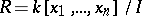.

A-derivation ofis a-linear mapping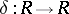that satisfies the Leibniz rule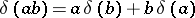for all pairs of elements of.

The set of all such mappings is a Lie algebra (often non-commutative; cf. also Commutative algebra) that is a finitely-generated-module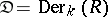. The algebra and module structures of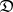often code aspects of the singularities of.

A more primitive object attached tois its module of Kähler differentials,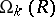, of whichis its-dual,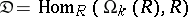.

More directly, the structure ofreflects many properties of. Thus, the classical Jacobian criterion asserts thatis a smooth algebra overexactly whenis a projective-module (cf. also Projective module).

For an algebrawithout non-trivial nilpotent elements, local complete intersections are also characterized by saying that the projective dimension of(cf. also Dimension) is at most one.

The technical issues linking these properties are the comparison between the set of polynomials that define, represented by the ideal, and the syzygies of eitheror(cf. also Syzygy).

The Zariski–Lipman conjecture makes predictions about, similar to those properties of.

The most important of these questions is as follows. Ifis-projective, thenis a regular ring (in commutative algebra). More precisely, it predicts that if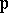is a prime ideal for whichis a free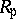-module, thenis a regular ring.

In [a3], the question is settled affirmatively for rings of Krull dimension(cf. also Dimension), and in all dimensions the rings are shown to be normal (cf. also Normal ring). Subsequently, G. Scheja and U. Storch [a4] established the conjecture for hypersurface rings, that is, whenis defined by a single equation,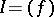.

As of 2000, the last major progress on the question was the proof by M. Hochster [a2] of the graded case.

A related set of questions is collected in [a5]: whether the finite projective dimension of eitherornecessarily forcesto be a local complete intersection. It is not known (as of 2000) whether this is true ifis projective, a fact which would be a consequence of the Zariski–Lipman conjecture. Several lower dimension cases are known, but the most significant progress was made by L. Avramov and J. Herzog when they solved the graded case [a1].

How to Cite This Entry:
Zariski-Lipman conjecture. Encyclopedia of Mathematics. URL: http://encyclopediaofmath.org/index.php?title=Zariski-Lipman_conjecture&oldid=16673
This article was adapted from an original article by W. Vasconcelos (originator), which appeared in Encyclopedia of Mathematics - ISBN 1402006098. See original article